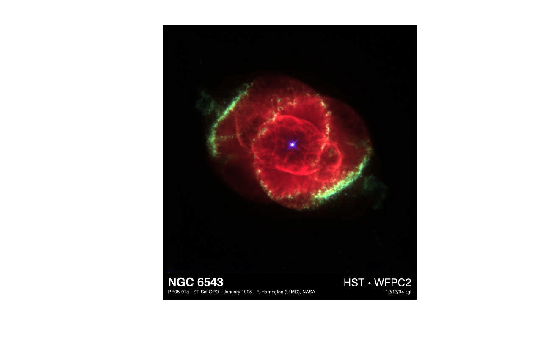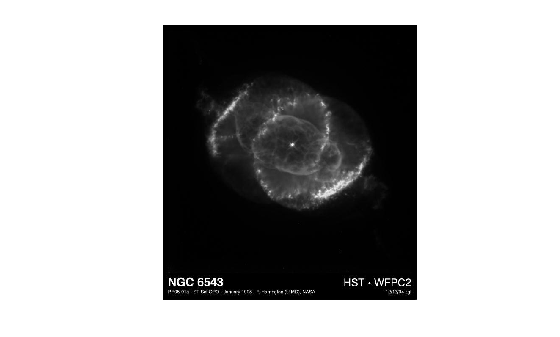im2gray

Convert RGB image to grayscale

Description

example

I = im2gray(RGB) converts the specified truecolor image RGB to a grayscale intensity image I. The im2gray function accepts grayscale images as inputs and returns them unmodified.

The im2gray function converts RGB images to grayscale by eliminating the hue and saturation information while retaining the luminance.

Examples

collapse all

Read a truecolor (RGB) image into the workspace from a file and display it.

imshow(RGB)Convert the RGB image into a grayscale image.

I = im2gray(RGB);

Display the converted grayscale image.

imshow(I)Input Arguments

collapse all

Truecolor image, specified as an m-by-n-by-3 numeric array. im2gray also accepts m-by-n numeric arrays (grayscale images) and returns them unmodified.

If you have Parallel Computing Toolbox™ installed, RGB can also be a gpuArray.

Data Types: single | double | uint8 | uint16

Output Arguments

collapse all

Grayscale image, returned as an m-by-n numeric array. If the input to im2gray is a grayscale image, the output image I is the same as the input image.

If you have Parallel Computing Toolbox installed, then I can also be a gpuArray.

Tips

• The im2gray function is identical to rgb2gray except that it can accept grayscale images as inputs, returning them unmodified. The rgb2gray function returns an error if the input image is a grayscale image. If you use the im2gray function, code like this loop is no longer necessary.

if ndims(I) == 3
I = rgb2gray(I);
end

• Unlike the rgb2gray function, the im2gray function does not accept colormaps as an input. To convert a colormap to grayscale, use the cmap2gray function.

Algorithms

The im2gray function converts RGB values to grayscale values by forming a weighted sum of the R, G, and B components:

0.2989 * R + 0.5870 * G + 0.1140 * B

These are the same weights used by the rgb2ntsc (Image Processing Toolbox) function to compute the Y component.

The coefficients used to calculate grayscale values in the im2gray function are identical to those used to calculate luminance (E'y) in Rec.ITU-R BT.601-7 after rounding to three decimal places.

Rec.ITU-R BT.601-7 calculates E'y using the following formula:

0.299 * R + 0.587 * G + 0.114 * B